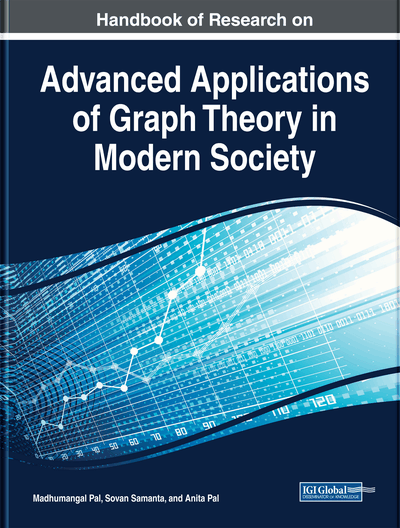# Tripartite and Quadpartite Size Ramsey Numbers for All Pairs of Connected Graphs on Four Vertices

Chula J. Jayawardene (University of Colombo, Sri Lanka)
DOI: 10.4018/978-1-5225-9380-5.ch010
Available
\$37.50
No Current Special Offers

## Abstract

A popular area of graph theory is based on a paper written in 1930 by F. P. Ramsey titled “On a Problem on Formal Logic.” A theorem which was proved in his paper triggered the study of modern Ramsey theory. However, his premature death at the young age of 26 hindered the development of this area of study at the initial stages. The balanced size multipartite Ramsey number mj (H,G) is defined as the smallest positive number s such that Kj×s→ (H,G). There are 36 pairs of (H, G), when H, G represent connected graphs on four vertices (as there are only 6 non-isomorphic connected graphs on four vertices). In this chapter, the authors find mj (H, G) exhaustively for all such pairs in the tripartite case j=3, and in the quadpartite case j=4, excluding the case m4 (K4,K4). In this case, the only known result is that m4 (K4,K4) is greater than or equal to 4, since no upper bound has been found as yet.
Chapter Preview
Top

## History

After the publication of the original paper by F. P. Ramsey, the resurrection of Ramsey Theory for graphs emerged in the paper by Paul Erdös and George Szekeres, published around 1935.

Theorem (by Paul Erdös and George Szekeres)

and

Following the publication of the paper by Paul Erdös and George Szekeres, the exact determination of these numbers for small graphs has been attempted by many mathematicians. Unfortunately, their progress was hindered by the stubbornly resistant (), which is presently known to lie between 43 and 48 (see Vigleik Angeltveit and Brendan D. McKay (Angelteveit, 2017) for the best upper bound of 48 and Exoo (Exoo, 1989) for the best lower bound of 43). Finding sharp bounds for () appears to be an even more arduous task. This has been eloquently expressed by the great mathematician Paul Erdös using the following quotation (see 1990 Scientific American article by Ronald Graham and Joel Spencer).Suppose aliens invade the earth and threaten to obliterate it in a year's time unless human beings can find the Ramsey number for red five and blue five (). We could marshal the world's best minds and fastest computers, and within a year we could probably calculate the value. If the aliens demanded the Ramsey number for red six and blue six (), however, we would have no choice but to launch a pre-emptive attack. – Paul Erdös (1990 Scientific American article by Ronald Graham & Joel Spencer)

Researchers are now trying to approach this problem by using new techniques to investigate whether the lower bound 43 (Exoo, 1989) and the upper bound 48 (Angelteveit (2017)) could be improved. Offshoots of classical Ramsey numbers, introduced by Burger and Vuuren (2004), Syfrizal et al., (2005, 2012), are the multipartite Ramsey numbers.

Let KN denote the complete graph on N vertices. For any two graphs, say H, G (without loops and parallel edges), we say that KN → (H,G), if for any red/blue colouring of KN, given by KN = HRHB, there exists a red copy H in HR or a blue copy G in HB. In Ramsey theory one important part deals with the exact determination of Ramsey numbers, r(H,G), defined as the smallest positive number N such that KN → (H,G). Formally, the balance multipartite graphcan be viewed as a graph consisting of the vertex set

and the edge set

## Complete Chapter List

Search this Book:
Reset